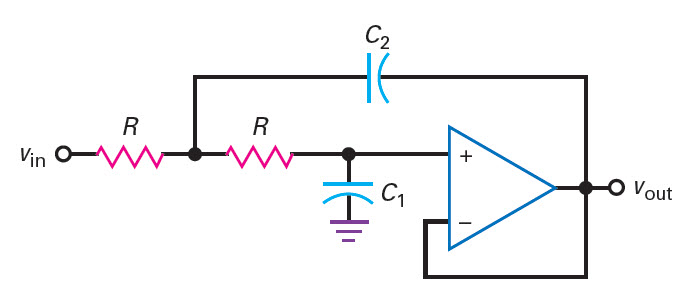# Sallen-Key 2nd Order Unity Gain LPF Design Calculator

The following is online calculator for Sallen-Key 2nd Order Unity Gain Low Pass Filter Design. In Sallen-Key 2nd Order Unity Gain LPF Design the two resistors are chosen equal. The two capacitors are unequal. The capacitor sets the Q of the filter. The passband voltage gain is unity(Av=1). Sallen key filters are VCVS filters because the op-amp is used as voltage controlled voltage source. With VCVS filters Butterworth, Chebyshev, and Bessel filters can be implemented.

Sallen-Key 2nd Order Unity Gain LPF Design Online Calculator
Inputs:

Formula Used:
$$A_v = 1, \\ Q=0.5\sqrt{\frac{C_2}{C_1}}, \\ f_p = \frac{1}{2 \pi R \sqrt{C_1 C_2}}, \\ f_c = K_c f_p \\ Butterworth:Q=0.707,K_c=1, \\ Bessel:Q=0.577,K_c=0.786$$Theoretical Results:

#### Notes on Sallen-Key 2nd Order Unity Gain LPF Design Calculator

• Voltage Gain
The voltage gain of this filter is unity.

$$A_v=1$$
• Quality Factor(Q)
The Q of the filter is given by,
$$Q=0.5\sqrt{\frac{C_2}{C_1}}$$
• Pole Frequency

The pole frequency is given by,

$$f_p = \frac{1}{2 \pi R \sqrt{C_1 C_2}}$$

• Cuttoff Frequency

The cutoff frequency is given by,

$$\\ f_c = K_c f_p$$

For Butterworth filter;

Q=0.707 and Kc=1

For Bessel filter;

Q=0.577 and Kc=0.786

•  Capacitor Values
For best result select the capacitors value in the range 0.001uF and 0.1uF.
• Rolloff
The rolloff of the second order filter is -40dB/decade. This is double of the first order active LPF circuit.

The Sallen-Key 2nd order filter is one of the popular 2nd order active filter. It uses single operational amplifier and two RC low pass filter in its construction. It is used as a basic building blocks to construct higher order filters. When odd order filter is to be constructed we can use first order active filter and the Sallen key 2nd order filter to form 3rd order filter. We can similarly cascade two 2nd order filter and single first order filter to form 5th order active filter.

The Sallen Key filter has advantage that it uses single op-amp and it has high input impedance and low output impedance. The filter stability is also good.